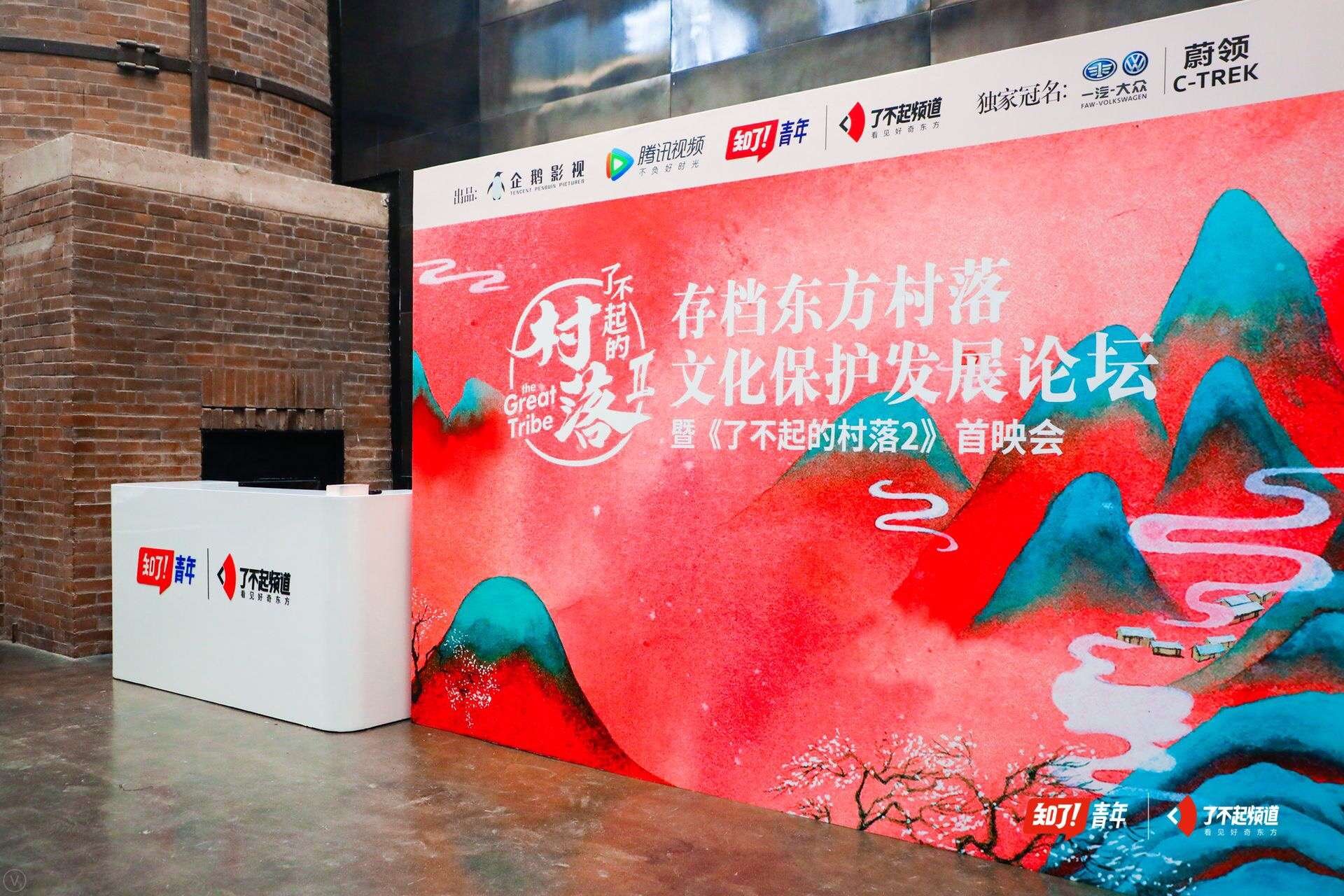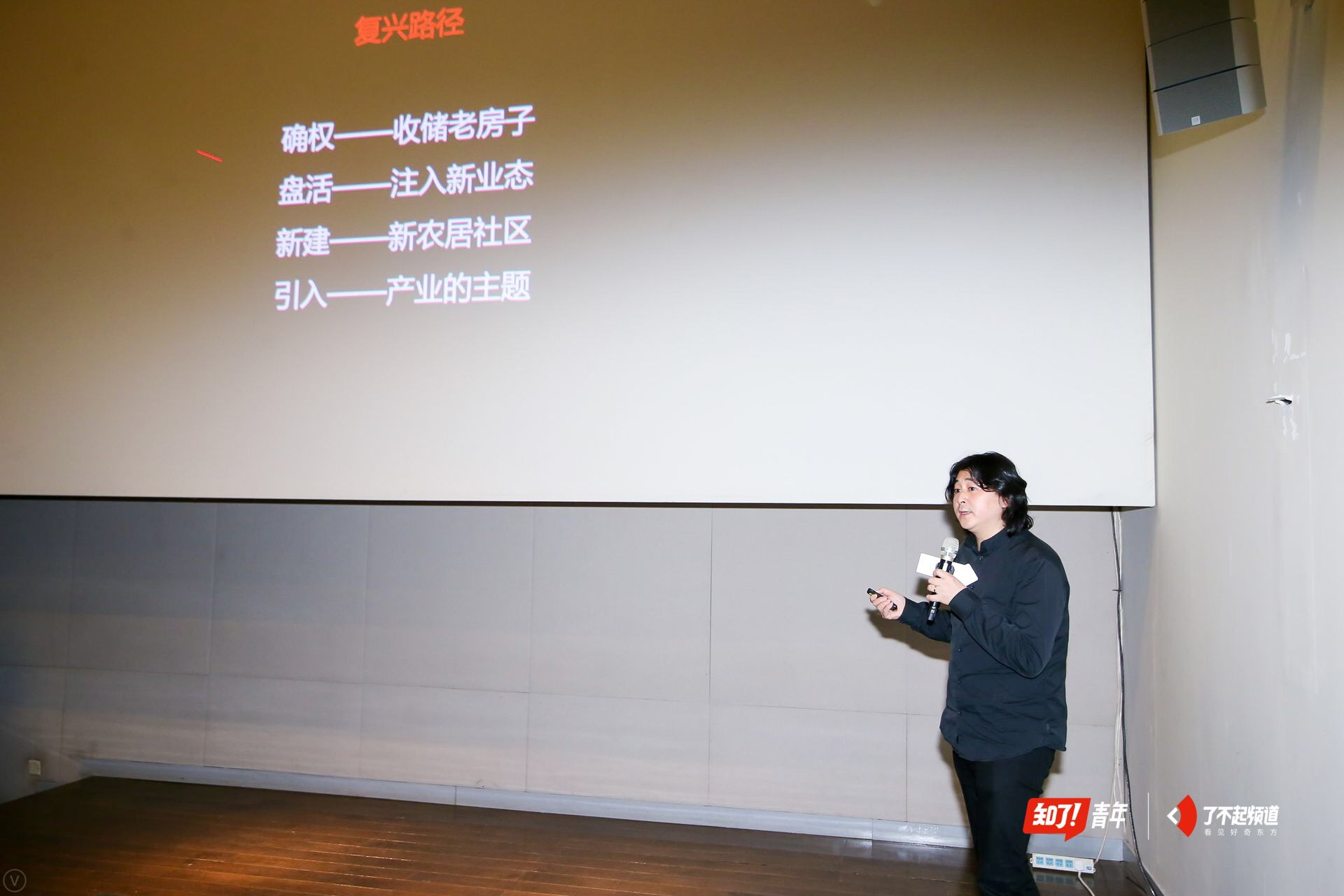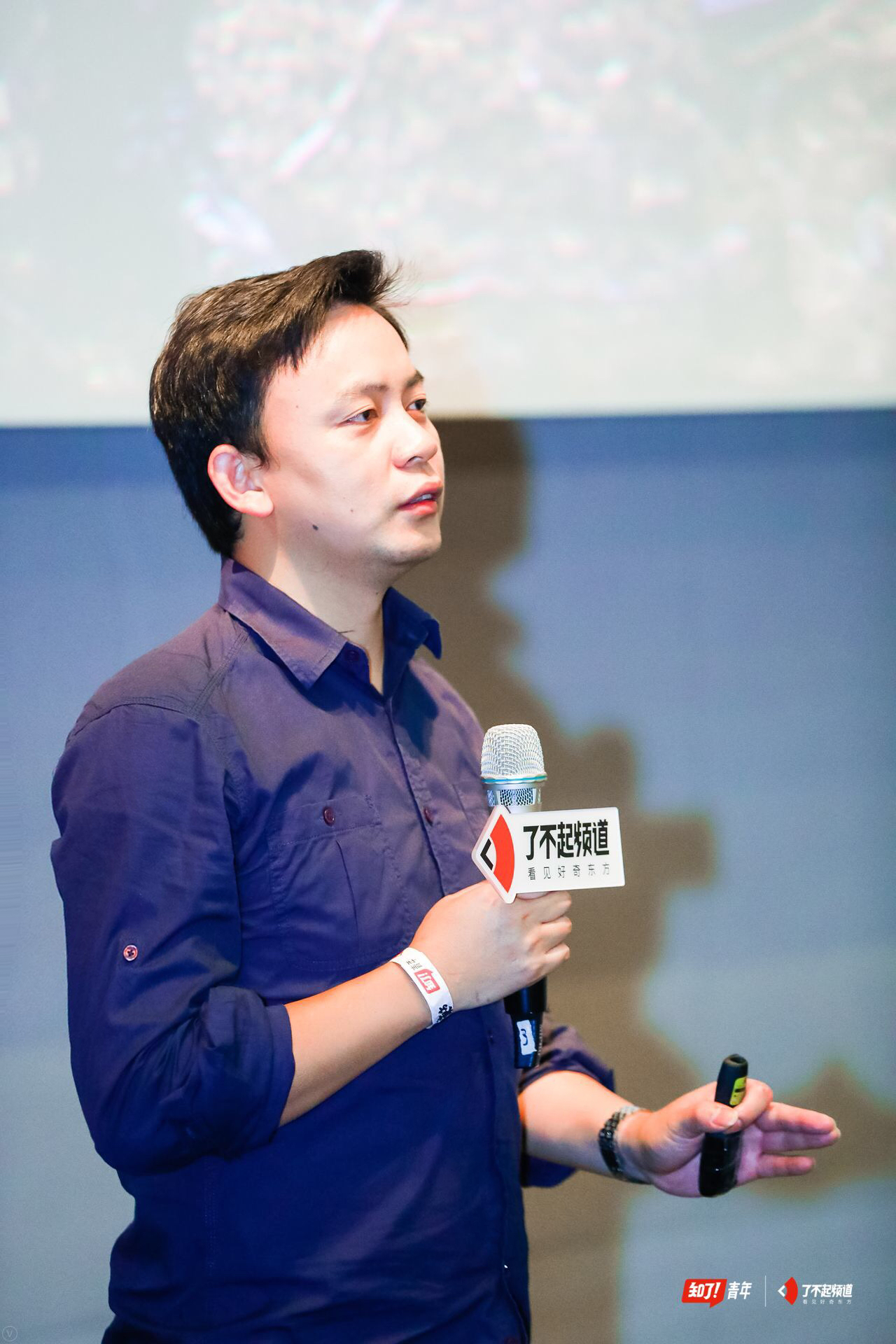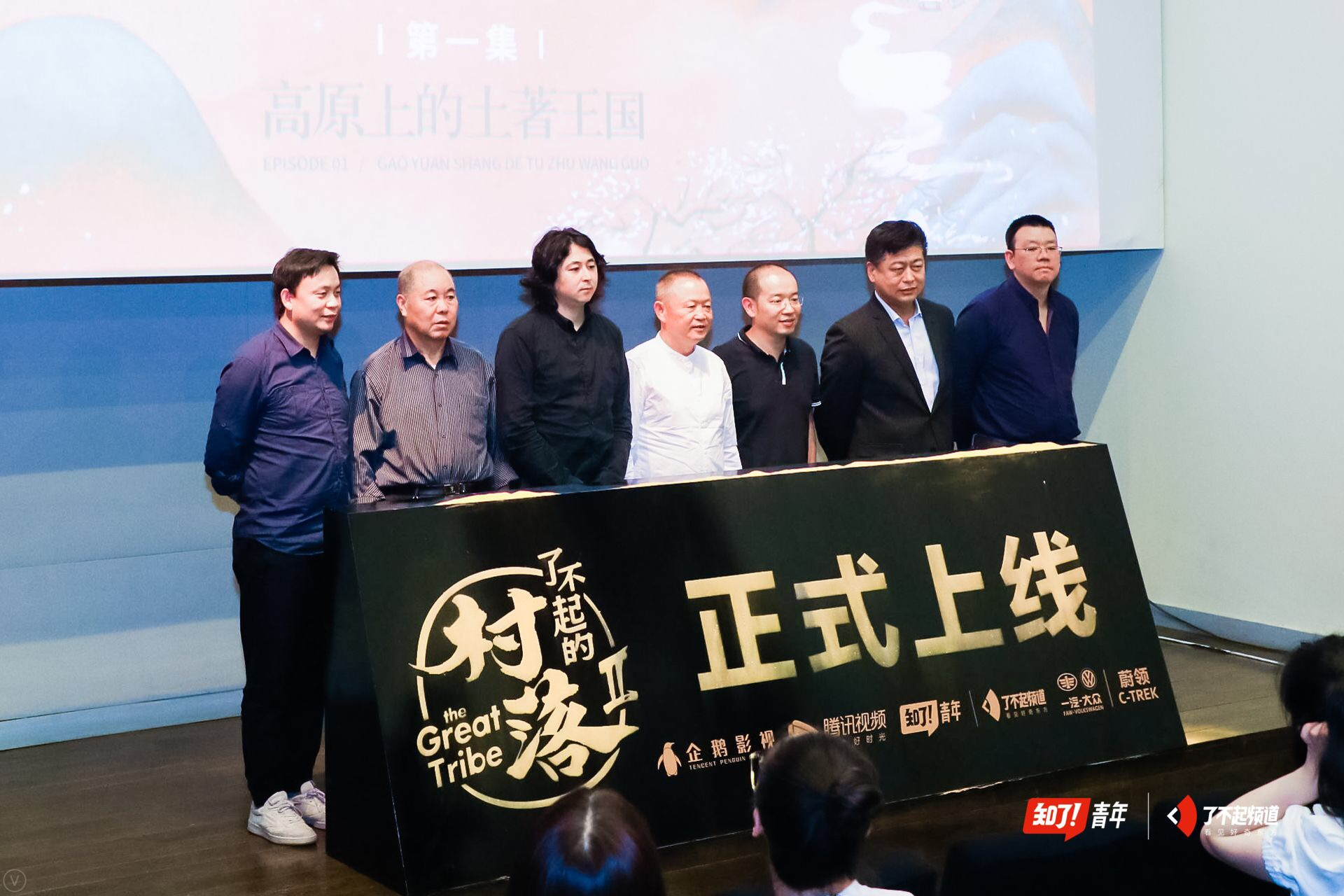|

# 了不起频道为村落发声 东方村落文化保护之旅在京启程

5月14日，存档东方村落文化保护发展论坛暨《了不起的村落2》寻色之旅季首映会在北京举行。本次活动旨在推动东方村落保护和农村未来发展，这场行业盛会吸引了知了青年（中国）文化有限公司CEO/了不起频道创始人李武望、中南大学二级教授/中国村落文化研究中心主任胡彬彬教授、中国国家地理图书出版人/地道风物CEO陈沂欢、新锐设计师/《了不起的村落1》东梓关村总设计师孟凡浩、房山区民间艺术家协会副主席/京西隗氐文化研究联谊会主席隗合显等专家及实践者参加，共同讨论东方村落的保护与发展。从村落出发，为村落发声

“结庐在人境，而无车马喧。”陶渊明悠然见得的南山，在经济高速发展的当下逐步城市化，一份繁荣取代了另一份美好。据统计，2000年中国共有村落360万个，到2017年，自然村只剩下不到200万个，10年间有90多万个村落在中国消失，平均每天20个。农民搬走了，村落空巢了，“一个人的村庄”已经不再是诗人的浪漫想象，而是村落日常记录的真实写照。

胡彬彬教授在论坛上强调：“传统村落因各种人为或自然的原因，正在以惊人的速度消失。与此伴生的是，传统村落原来所具有的代代相传、传承至今的文化形态正在发生急剧裂变，传统文化的内容在结构也面临着支离破碎的危险。”村落文化的存档迫在眉睫。伴随经济和社会发展,传统村落的凋敝没落成为普遍趋势，然而传统村落中包含着历史形成的，具有唯一性的传统价值和记忆秩序，如果简单地以新代旧，寻求物质利益和功能的满足，将会消解历史形成的文化主体感、认同感、凝聚力和特色文化资源。

对于村落的重生，此次与会专家之一——新锐设计师/《了不起的村落1》东梓关村总设计师孟凡浩感触颇深。东梓关村原本是杭州市一个不起眼的村庄，虽然拥有独特历史文化秉赋，但无论是自然资源、还是知名度都比不上与其相邻的龙门古镇，尤其是水陆交通的衰落，导致东梓关村彻底地萧条落寞。然而现在，东梓关村通过重新设计，恢复了村落的原真性与多样化的场所感，再造了当代村落，使村子重新焕发了勃勃生机。现场东梓关村项目的总设计师孟凡浩以东梓关村的实践经验为例，解密了他如何为村落的非物质文化遗产赋新，让传统文化重新焕发生机，引发了对于村落内核牵引长期驱动力的思考。村落100探访计划 了不起频道开启寻色之旅

“了不起频道”用时代切口，以年轻的表达式探索，以新一代纪录片的方式呈现并解读东方奥秘，通过那些了不起的人和物，见识新鲜有创造力“本真东方”，致力于打造中国年轻一代的垂直文化媒体。目前，了不起频道的内容矩阵已搭载了《了不起的匠人》、《了不起的村落》、《三日为期》等多部高品质东方文化纪实类IP，入驻40多家平台，在全网平台粉丝数超过600万，单条视频播放量超3000万，全平台视频播放量超20亿。

针对村落文化逐渐消逝的社会文化现象，了不起频道开启了“100个村落”探访计划，旨在用影像纪录100个村落的美好，唤回大众对东方村落的关注，让那些在城市化大潮下濒临消逝的村落有迹可循。为了村落100探访计划，团队整理了326特色村落的选题，探访了18个村落，采访了200多位村民，一共跑了33762千米，最北去到了内蒙根河的敖鲁古雅，最西北到了新疆喀纳斯的禾木，最南去到了海南的儋州，最东去到了福建霞浦，致力于用5年的时间，对国内百座村落进行存档，用一百次抨击心灵的本真记录，留给后世一本关于村落的百科全书。

“印象最深的是去年第一季驯鹿村的柳霞一家，当时第一次去调研时与柳霞的丈夫老翟相聊甚欢，然而一个月后就在我们出发拍摄前夕，得知老翟已心脏病发不幸去世。拍摄前两天前往柳霞家复景时，柳霞家的鹿都还在，隔了一天，大队人马赶往拍摄，却发现她家的鹿竟然在一天时间里跑得一头不剩。”知了青年（中国）文化有限公司CEO/了不起频道创始人李武望回忆道，“再过三十年，也许大兴安岭就不会再有鄂温克的养鹿人了，驯鹿村将不复存在。我们希望在这些村落消逝之前，留下一个备忘录来与过去促膝长谈，所以我们决定：要用我们擅长的方式，存档100个东方村落。”《了不起的村落1》记录了神州大地的10个村落，从大兴安岭连绵起伏的驯鹿村，到地处晋蒙交界的黄河古村老牛湾村，观众跟随着摄制组的镜头一起上山下海，为存档地图上散落的文明横跨中华大地。由一汽-大众蔚领独家冠名的《了不起的村落2》寻色之旅季依然是村落100探访计划，以“来自山川湖海，偏爱本真初心”来作全新表达，以传统东方的色彩为引子，纵横东西南北中，探索了12个奇观地貌下的极致村落，联系自然地貌及村落个性，将全部重心落在了寻找自然环境下人们最本真的生活状态以及动人的细节故事之上，寻找多彩胜境中的人间烟火。

《了不起的村落2》寻色之旅季由一汽-大众蔚领与了不起频道、腾讯视频、企鹅影视联合荣誉呈现。此次存档东方村落文化保护发展论坛由知了青年（中国）文化有限公司首席内容官熊熠主持，知了青年CEO李武望、一汽-大众销售有限责任公司副总经理马振山、企鹅影视纪录片工作室总监朱乐贤也莅临了存档东方村落文化保护发展论坛暨《了不起的村落2》寻色之旅季首映会现场，助力村落文化的保护与发展。对于冠名，一汽-大众销售有限责任公司副总经理马振山称：“《了不起的村落》与蔚领在品牌主张与理念上高度契合，我们也希望，未来能有更多的人在一汽-大众蔚领的带领下，去发现、去感受、去传承东方村落独有的传统与文化。”“令人欣慰的是，能有这么多的观众被村落的故事所打动，开始关注村落100计划，也有观众开始主动为我们推介村落。《了不起的村落2》寻色之旅季探寻分布于中国版图上的各种极致地貌里的村落，从这些村落里找到富有性格特征的东方色彩，也许能给我们的生活带来更多的启迪和灵感。”李武望在现场发出感慨。2016年起，了不起频道陆续推出了《了不起的匠人》三季、《了不起的村落》、《三日为期》等一系列年轻态、创新型纪录片，一反传统的思维模式，充分发挥文化力+设计力+运营力，通过文化产品营销包打通用户、客户、线上与线下，得到了市场认可。今年，这群了不起频道原班团队再次打造的新一代纪录片——《了不起的村落2》寻色之旅季又会为新生代文化内容带来哪些惊喜？据悉，《了不起的村落2》寻色之旅季将于5月15日在腾讯视频全网独播，初心不变，使命仍在。

`声明：本文由入驻焦点开放平台的作者撰写，除焦点官方账号外，观点仅代表作者本人，不代表焦点立场错误信息举报电话： 400-099-0099，邮箱：jubao@vip.sohu.com，或点此进行意见反馈，或点此进行举报投诉。`A B C D E F G H J K L M N P Q R S T W X Y Z
A - B - C - D - E
• A
• 鞍山
• 安庆
• 安阳
• 安顺
• 安康
• 澳门
• B
• 北京
• 保定
• 包头
• 巴彦淖尔
• 本溪
• 蚌埠
• 亳州
• 滨州
• 北海
• 百色
• 巴中
• 毕节
• 保山
• 宝鸡
• 白银
• 巴州
• C
• 承德
• 沧州
• 长治
• 赤峰
• 朝阳
• 长春
• 常州
• 滁州
• 池州
• 长沙
• 常德
• 郴州
• 潮州
• 崇左
• 重庆
• 成都
• 楚雄
• 昌都
• 慈溪
• 常熟
• D
• 大同
• 大连
• 丹东
• 大庆
• 东营
• 德州
• 东莞
• 德阳
• 达州
• 大理
• 德宏
• 定西
• 儋州
• 东平
• E
• 鄂尔多斯
• 鄂州
• 恩施
F - G - H - I - J
• F
• 抚顺
• 阜新
• 阜阳
• 福州
• 抚州
• 佛山
• 防城港
• G
• 赣州
• 广州
• 桂林
• 贵港
• 广元
• 广安
• 贵阳
• 固原
• H
• 邯郸
• 衡水
• 呼和浩特
• 呼伦贝尔
• 葫芦岛
• 哈尔滨
• 黑河
• 淮安
• 杭州
• 湖州
• 合肥
• 淮南
• 淮北
• 黄山
• 菏泽
• 鹤壁
• 黄石
• 黄冈
• 衡阳
• 怀化
• 惠州
• 河源
• 贺州
• 河池
• 海口
• 红河
• 汉中
• 海东
• 怀来
• I
• J
• 晋中
• 锦州
• 吉林
• 鸡西
• 佳木斯
• 嘉兴
• 金华
• 景德镇
• 九江
• 吉安
• 济南
• 济宁
• 焦作
• 荆门
• 荆州
• 江门
• 揭阳
• 金昌
• 酒泉
• 嘉峪关
K - L - M - N - P
• K
• 开封
• 昆明
• 昆山
• L
• 廊坊
• 临汾
• 辽阳
• 连云港
• 丽水
• 六安
• 龙岩
• 莱芜
• 临沂
• 聊城
• 洛阳
• 漯河
• 娄底
• 柳州
• 来宾
• 泸州
• 乐山
• 六盘水
• 丽江
• 临沧
• 拉萨
• 林芝
• 兰州
• 陇南
• M
• 牡丹江
• 马鞍山
• 茂名
• 梅州
• 绵阳
• 眉山
• N
• 南京
• 南通
• 宁波
• 南平
• 宁德
• 南昌
• 南阳
• 南宁
• 内江
• 南充
• P
• 盘锦
• 莆田
• 平顶山
• 濮阳
• 攀枝花
• 普洱
• 平凉
Q - R - S - T - W
• Q
• 秦皇岛
• 齐齐哈尔
• 衢州
• 泉州
• 青岛
• 清远
• 钦州
• 黔南
• 曲靖
• 庆阳
• R
• 日照
• 日喀则
• S
• 石家庄
• 沈阳
• 双鸭山
• 绥化
• 上海
• 苏州
• 宿迁
• 绍兴
• 宿州
• 三明
• 上饶
• 三门峡
• 商丘
• 十堰
• 随州
• 邵阳
• 韶关
• 深圳
• 汕头
• 汕尾
• 三亚
• 三沙
• 遂宁
• 山南
• 商洛
• 石嘴山
• T
• 天津
• 唐山
• 太原
• 通辽
• 铁岭
• 泰州
• 台州
• 铜陵
• 泰安
• 铜仁
• 铜川
• 天水
• 天门
• W
• 乌海
• 乌兰察布
• 无锡
• 温州
• 芜湖
• 潍坊
• 威海
• 武汉
• 梧州
• 渭南
• 武威
• 吴忠
• 乌鲁木齐
X - Y - Z
• X
• 邢台
• 徐州
• 宣城
• 厦门
• 新乡
• 许昌
• 信阳
• 襄阳
• 孝感
• 咸宁
• 湘潭
• 湘西
• 西双版纳
• 西安
• 咸阳
• 西宁
• 仙桃
• 西昌
• Y
• 运城
• 营口
• 盐城
• 扬州
• 鹰潭
• 宜春
• 烟台
• 宜昌
• 岳阳
• 益阳
• 永州
• 阳江
• 云浮
• 玉林
• 宜宾
• 雅安
• 玉溪
• 延安
• 榆林
• 银川
• Z
• 张家口
• 镇江
• 舟山
• 漳州
• 淄博
• 枣庄
• 郑州
• 周口
• 驻马店
• 株洲
• 张家界
• 珠海
• 湛江
• 肇庆
• 中山
• 自贡
• 资阳
• 遵义
• 昭通
• 张掖
• 中卫

1室1厅1厨1卫1阳台

1
2
3
4
5

0
1
2

1

1

0
1
2
3报名成功，资料已提交审核A B C D E F G H J K L M N P Q R S T W X Y Z
A - B - C - D - E
• A
• 鞍山
• 安庆
• 安阳
• 安顺
• 安康
• 澳门
• B
• 北京
• 保定
• 包头
• 巴彦淖尔
• 本溪
• 蚌埠
• 亳州
• 滨州
• 北海
• 百色
• 巴中
• 毕节
• 保山
• 宝鸡
• 白银
• 巴州
• C
• 承德
• 沧州
• 长治
• 赤峰
• 朝阳
• 长春
• 常州
• 滁州
• 池州
• 长沙
• 常德
• 郴州
• 潮州
• 崇左
• 重庆
• 成都
• 楚雄
• 昌都
• 慈溪
• 常熟
• D
• 大同
• 大连
• 丹东
• 大庆
• 东营
• 德州
• 东莞
• 德阳
• 达州
• 大理
• 德宏
• 定西
• 儋州
• 东平
• E
• 鄂尔多斯
• 鄂州
• 恩施
F - G - H - I - J
• F
• 抚顺
• 阜新
• 阜阳
• 福州
• 抚州
• 佛山
• 防城港
• G
• 赣州
• 广州
• 桂林
• 贵港
• 广元
• 广安
• 贵阳
• 固原
• H
• 邯郸
• 衡水
• 呼和浩特
• 呼伦贝尔
• 葫芦岛
• 哈尔滨
• 黑河
• 淮安
• 杭州
• 湖州
• 合肥
• 淮南
• 淮北
• 黄山
• 菏泽
• 鹤壁
• 黄石
• 黄冈
• 衡阳
• 怀化
• 惠州
• 河源
• 贺州
• 河池
• 海口
• 红河
• 汉中
• 海东
• 怀来
• I
• J
• 晋中
• 锦州
• 吉林
• 鸡西
• 佳木斯
• 嘉兴
• 金华
• 景德镇
• 九江
• 吉安
• 济南
• 济宁
• 焦作
• 荆门
• 荆州
• 江门
• 揭阳
• 金昌
• 酒泉
• 嘉峪关
K - L - M - N - P
• K
• 开封
• 昆明
• 昆山
• L
• 廊坊
• 临汾
• 辽阳
• 连云港
• 丽水
• 六安
• 龙岩
• 莱芜
• 临沂
• 聊城
• 洛阳
• 漯河
• 娄底
• 柳州
• 来宾
• 泸州
• 乐山
• 六盘水
• 丽江
• 临沧
• 拉萨
• 林芝
• 兰州
• 陇南
• M
• 牡丹江
• 马鞍山
• 茂名
• 梅州
• 绵阳
• 眉山
• N
• 南京
• 南通
• 宁波
• 南平
• 宁德
• 南昌
• 南阳
• 南宁
• 内江
• 南充
• P
• 盘锦
• 莆田
• 平顶山
• 濮阳
• 攀枝花
• 普洱
• 平凉
Q - R - S - T - W
• Q
• 秦皇岛
• 齐齐哈尔
• 衢州
• 泉州
• 青岛
• 清远
• 钦州
• 黔南
• 曲靖
• 庆阳
• R
• 日照
• 日喀则
• S
• 石家庄
• 沈阳
• 双鸭山
• 绥化
• 上海
• 苏州
• 宿迁
• 绍兴
• 宿州
• 三明
• 上饶
• 三门峡
• 商丘
• 十堰
• 随州
• 邵阳
• 韶关
• 深圳
• 汕头
• 汕尾
• 三亚
• 三沙
• 遂宁
• 山南
• 商洛
• 石嘴山
• T
• 天津
• 唐山
• 太原
• 通辽
• 铁岭
• 泰州
• 台州
• 铜陵
• 泰安
• 铜仁
• 铜川
• 天水
• 天门
• W
• 乌海
• 乌兰察布
• 无锡
• 温州
• 芜湖
• 潍坊
• 威海
• 武汉
• 梧州
• 渭南
• 武威
• 吴忠
• 乌鲁木齐
X - Y - Z
• X
• 邢台
• 徐州
• 宣城
• 厦门
• 新乡
• 许昌
• 信阳
• 襄阳
• 孝感
• 咸宁
• 湘潭
• 湘西
• 西双版纳
• 西安
• 咸阳
• 西宁
• 仙桃
• 西昌
• Y
• 运城
• 营口
• 盐城
• 扬州
• 鹰潭
• 宜春
• 烟台
• 宜昌
• 岳阳
• 益阳
• 永州
• 阳江
• 云浮
• 玉林
• 宜宾
• 雅安
• 玉溪
• 延安
• 榆林
• 银川
• Z
• 张家口
• 镇江
• 舟山
• 漳州
• 淄博
• 枣庄
• 郑州
• 周口
• 驻马店
• 株洲
• 张家界
• 珠海
• 湛江
• 肇庆
• 中山
• 自贡
• 资阳
• 遵义
• 昭通
• 张掖
• 中卫• 手机• 分享
• 设计
免费设计
• 计算器
装修计算器
• 入驻
合作入驻
• 联系
联系我们
• 置顶
返回顶部